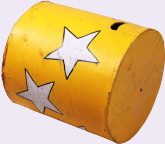# How To Calculate The Area Of A CylinderUnderstanding how to find the area of a cylinder is easy if one first visualizes the cylinder and breaks its surface down into component pieces. To do this, first take a good look at the most common cylinder encountered in life: the toilet paper roll. Use a pair of scissors to cut one open and you will see that it is just a rectangular piece of cardboard that can be rolled up so that its edges meet. The ends are just two identical circles. From this little exercise it becomes clear that the surface area of a cylinder is just the sum of the area of the rolled up rectangle that makes the body and the area of the two identical circles. Wait a minute! You can't just unroll a cylinder and pop off the ends to measure them, can you? Well, no...but you can do the arithmetic as though that is what happened, by using the properties of a circle.

In a circle, the distance around the edge is called the circumference. If you think about that rectangle that got rolled up to make the cylinder, you will realize that the circumference of the cylinder is exactly equal to the length of the unjoined sides. The height (or length) of the cylinder is equal to the length of the joined sides. You still can't unroll the cylinder, but as long as you know or can determine the circumference of the cylinder, you don't have to unroll it. The circumference is related to the distance from the centerline of the cylinder to its outside edge by the factor of 2p, and the length of the cylinder is just the length of the cylinder. So the surface area of the body of the cylinder is obtained by multiplying these values together. The two ends of the cylinder are no more than circles and the area of each one is given by multiplying the radius by itself and then by the value of P.

The total surface area of a cylinder is then simply calculated by adding together the three individual areas of the body and the two ends, according to the general formula A = (2p X r X l) + (p X r2) + (p X r2), or A = 2prl + 2pr2, or A = 2p(rl + r2). As an example of how to use this, imagine you have a large tank that has to be painted a certain color. You have enough of the paint to cover 150 square feet, and you want to know if you will need more. The tank is 11 feet long and 5 feet wide, which makes the radius 2.5 feet. The total area is found by substituting these values into the formula. (Use the value of p to be 3.14, close enough for most practical applications). A = 2p(rl + r2), = 2p X ((2.5 X 11) + (2.5 X 2.5)), = 6.28 X (27.5 + 6.25), = 6.28 X 33.75, = 211.95 square feet. Go get more paint.

Richard M J Renneboog, MSRichard M. J. Renneboog is an independent private technical consultant and writer in both chemical and computer applications. Endeavors have included preparation of scripts for instructional and promotional video, corporate website design, curriculum development for training in advanced composites technology, and development.

### Science Articles: